# Profit, Cost, and Revenue Functions

Coming to Statistica
in 2016/2017

Group Classes

Interactive Webinars

Blog

Guestbook.

E-mail     anne@statistica.com.au

# Place         Willetton, Western Australia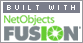Revenue Function, R(x)             Total income from producing units. Cost Function, C(x)                      Total cost of producing the units.Profit Function, P(x)                   Total Income minus Total Cost. Profit = Income -    Cost  P(x)   =     R(x)   -    C(x) Marginal is rate of change of cost, revenue or profit with the respect to the number of units. This means differentiate the cost, revenue or profit.    Marginal Revenue, R'(x)                                 The derivative of R(x). Marginal Cost Function, C'(x)                      The derivative of C(x). Marginal Profit Function, P'(x)                   The derivative of P(x).   In words, the word “Marginal” can be read as “the next unit”, Marginal Revenue, R'(x)                    The next unit will make this Revenue Marginal Cost Function, C'(x)         The next unit will be this Cost Marginal Profit Function, P'(x)      The next unit will make this ProfitQuestionA manufacturing company  produces and sells tables. The cost function is given by: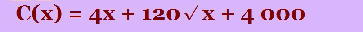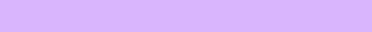where x is the  number of tables.   The tables are  sold for \$200 each. Find 1. The total cost of producing 25 tables. 2. The total revenue and total profit from selling 25 tables. 3. The approximate cost of producing the 201st table. 4. The approximate profit on the next table after selling 200 tables . 5. The average cost per table of 200 tables.Total cost of producing 25 tables It really is simple, put 25 wherever you see x  in C(x).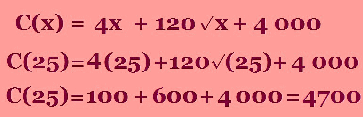Now you know me, I like that little sentence at the end. Just use the question to help you write the  sentence. The total cost of  producing 25 tables is \$4700. The approximate cost of producing the 201st table So we want to know after making 200 tables, how much does it cost  to produce one more table.  Another way this could have been written is after producing 200 tables, how much does it cost for the next table. So read the words very  carefully. We will have to differentiate C(x)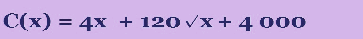C(x) = 4x + 120  x (1/2) + 4000 C'(x) = 4 +  120 (1/2)  x (1/2 - 1) + 0  This is simple  differentiation C'(x) = 4  +  60 x - (1/2) Now the x-value  that we are going to use is 200 as the next table will be 201st. C'(x) = 4  + 60(  200 - (1/2) ) C'(x) = 4  +  60(0.0707) C'(x) = 4  + 4.242   =  8.242 The approximate cost of  making the 201st table is \$8.24.Total revenue and total profit from selling 25 tables Revenue is Income, Cost is expense and the difference (Revenue - Cost)  is Profit or Loss. So the Revenue is the amount you sell the tables for multiplied by how many tables.  Once again put  x = 25 R(x) =  200 x = 200(25) =   5000 P(x) = R(x) - C(x)            = 5000 - 4700  = 300 (4700 came from part 1) Remember those  sentences: The total revenue from selling 25 tables is \$5000. The total profit from selling 25 tables is \$300. The approximate profit on the next table after selling 200 tables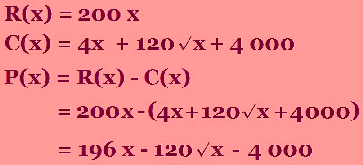Now we see the word approximate and we think differentiate and put 200 in for the value of x. Remember 201 would be the next number after 200. P'(x) = 196 - 60 x - (1/2) When x = 200,           P'(x) = 196 - 60(  200 - (1/2) )            =  196 -  60 (0.0707) P'(x) = 196 - 4.242 =  191.758 The approximate profit on the next table after 200 tables have been sold is  \$191.76.The average cost per table of 200 tables This is just like any other average so find the cost for 200 tables and then divide by 200. We will have to differentiate C(x)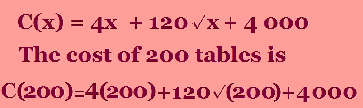C(200) = 800 + 120(14.1421) +4000 = \$6497.06 The average cost is  dividing this amount by 200. Average Cost (200) =  6497.06 / 200   = \$32.49 The average cost per table of 200 tables is \$32.49.[Home] [Parents] [Contact us] [Services] [Mathematics] [Statistics] [School]